# Car down

Sarah buys a car costing $12,500. It depreciates value by 8% in the first year, 10% in the second year, and 5% in the third year. Calculate the value of a car after the third year. ### Correct answer: x = 9832.5 ### Step-by-step explanation: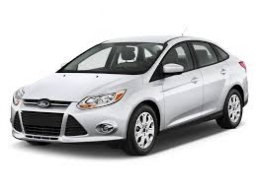Did you find an error or inaccuracy? Feel free to write us. Thank you! Showing 1 comment:Dr Math The original question was: Calculate what percent is the sales tax. Answer: I now cannot calculate any tax due I don't know your tax law codes etc.etc....Tips to related online calculators Our percentage calculator will help you quickly calculate various typical tasks with percentages. Do you want to convert time units like minutes to seconds? #### You need to know the following knowledge to solve this word math problem: ## Related math problems and questions: • Sales offIf a sweater sells for$ 19 after a 5% markdown, what was its original price?
• Saving 9An amount of $2000 is invested at an interest of 5% per month. If$ 200 is added at the beginning of each successive month but no withdrawals. Give an expression for the value accumulated after n months. After how many months will the amount has accumula
• Railways 3Railway Corporation wants to purchase a new machine for $360,000. Management predicts that the machine can produce sales of$220,000 each year for the next 5 years. Expenses are expected to include direct materials, direct labor, and factory overhead (exc
• Ice skates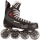Ice skates were raised twice, the first time by 25%, the second time by 10%. After the second price, their cost was 82.5 euros. What was the original price of skates?
• Sale offThe TV went down 10% and then 10% off the original price again. Now it costs 300 €. What was its original price?
• Nelly 2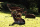Nelly buys a board game. She pays for the board game and pays 1.54 dollars in sales tax. The sales tax rate is 5.5 percent. What is the original price of the board game, before tax?
• Reducing balance method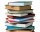A company buys an item having a useful life of 10 years for 1,000,000. If the company depreciates the item by the reducing balance method, a. Determine the depreciation for the first year. b. Estimate the depreciation for the second and third years. c. Wh
• Investment1000$is invested at 10% compound interest. What factor is the capital multiplied by each year? How much will be there after n=12 years? • Tabitha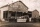Tabitha manufactures a product that sells very well. The capacity of her facility is 241,000 units per year. The fixed costs are$122,000 per year, and the variable costs are $11 per unit. The product currently sells for$17. a. What total revenue is requ
• Drilling machine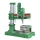A manufacturing firm purchased a heavy duty drilling machine. They were given two payment options: Option 1: Make a payment of $46,000 immediately to settle the invoice for the machine. Option 2: Make a payment of$21,500 immediately and the balance of $2 • Compound interest 3After 8 years, what is the total amount of a compound interest investment of$25,000 at 3% interest, compounded quarterly? (interest is now dream - in the year 2019)
• 3y inflation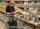Price of the roll rise in the first year by 9%, the second year fell by 5% and in the third year increased by 3%. Calculate the average annual increase in price of the roll.
• Two shopsIn two different shops, the same skis had the same price. In the first, however, they first became more expensive by 20% and then cheaper by 5%. In the second, they were first cheaper by 5% and then more expensive by 30%, so after these adjustments in the
• Car valueThe car loses value 15% every year. Determine a time (in years) when its price will halve.
• Exchange ratesIf the Canadian dollar appreciated by C$0.005 relative to the US dollar, what would be the new value of the Canadian dollar per US dollar? Assume the current exchange rate was US$1 = C\$0.907.
• InflationOnce upon a time, tsar owned a money printer and printed and printed. The result of printing money prices went up,in the first year 3.9 %, in the second 6%, in the third 4.7% and in the fourth 5.5%. Then tsar was failed in election. Calculate the average
• MerchantThe merchant lower cost by 10% in December when it was not sold. Again in January lower cost by 20% and now costs 576 €. A. How much the goods stood originally? B, how much cost the goods after the first lowering? C, How many percents totally merchant low